# Draw different pairs of circles. How many points does each pair have in common? What is the maximum number of common points?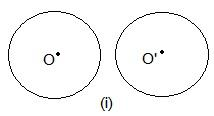In these two circles, there is no point in common.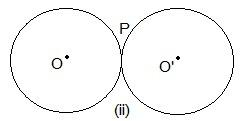Here, only one point “P” is common.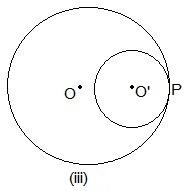Even here, P is the common point.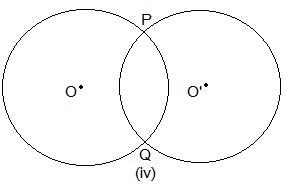Here, two points are common which are P and Q.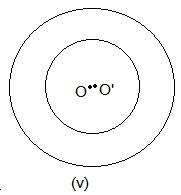No point is common in the above circle.